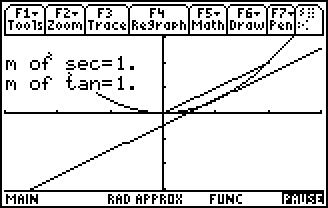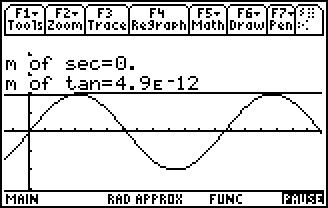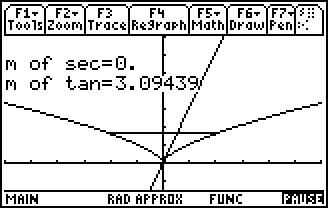# Activities

••• ##### Subject Area

• Math: Calculus: Applications of the Derivative

• ##### Author9-12

15 Minutes

• ##### Device
• TI-89 / TI-89 Titanium

TI Connect™

• ##### Accessories

TI Connectivity Cable

## Somewhere in the Middle#### Activity Overview

Students find out when the slope of a tangent line is parallel to the secant line passing through the endpoints of an interval to help them find the values of c guaranteed to exist by the Mean Value Theorem.

#### Key Steps

•Students are introduced to the Mean Value Theorem.

•Students use the tangent and secant lines to find the value of c for functions that have one or more values for c, or have no value for c.

•Then students try to use the tangent and secant lines to find the value of c for functions that have no value for c.

In an extension, students apply the Mean Value Theorem to prove that two horses had the same velocity during a race.